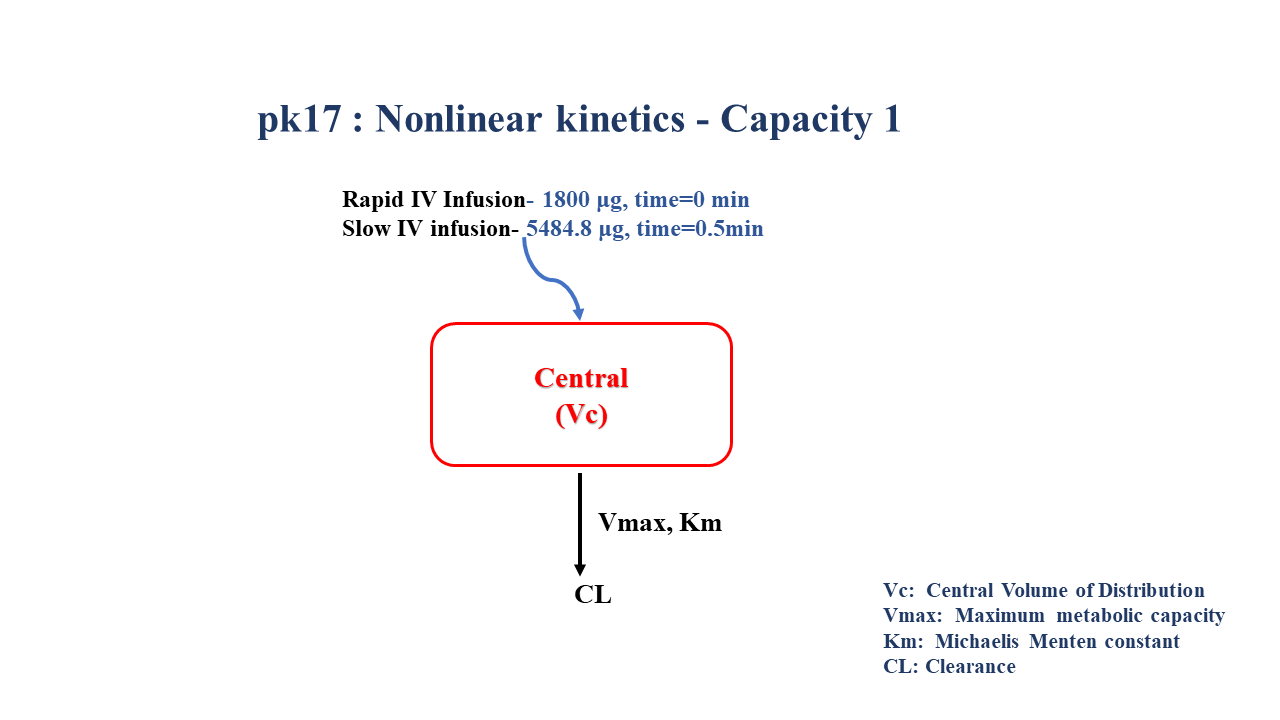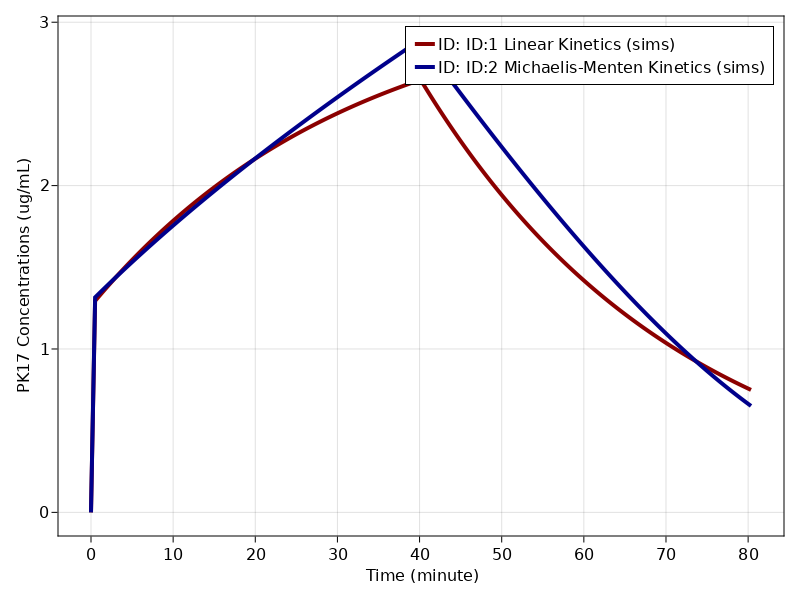# Exercise PK17 - Nonlinear kinetics - Capacity 1

### Background

Following info

• Structural model - One compartment non linear elimination

• Route of administration - IV infusion

• Dosage Regimen - 1800 μg Rapid IV, 5484.8 μg Slow IV

• Number of Subjects - 1### Learning Outcomes

In this model, you will learn -

• To build plasma concentration data following multiple intravenous infusions.

• To apply differential equation for one compartment model taking into consideration non linear elimination parameters.

### Objectives

In this tutorial, you will learn how to build one compartment model for capacity limited kinetics and to simulate the model for single subject following multiple IV infusions.

### Libraries

Call the "necessary" libraries to get started.

using Random
using Pumas
using PumasUtilities
using CairoMakie


### Model

In this One compartment model following linear elimination,multiple IV infusions are administered into the central compartment.

##### Linear Model
pk_17_lm     = @model begin
desc = "Linear One Compartment Model"
timeu = u"minute"
end

@param begin
"Clearance (ml/min)"
tvcl     ∈ RealDomain(lower=0)
"Volume of Distribution (ml)"
tvvc     ∈ RealDomain(lower=0)
Ω        ∈ PDiagDomain(2)
σ        ∈ RealDomain(lower=0)
end

@random begin
η        ~ MvNormal(Ω)
end

@pre begin
Cl       = tvcl   * exp(η)
Vc       = tvvc   * exp(η)
end

@dynamics begin
Central' = - (Cl/Vc)*Central
end

@derived begin
cp       = @. Central/Vc
"""
Observed Concentration (ug/ml)
"""
dv       ~ @. Normal(cp,σ)
end
end

PumasModel
Parameters: tvcl, tvvc, Ω, σ
Random effects: η
Covariates:
Dynamical variables: Central
Derived: cp, dv
Observed: cp, dv

##### Parameters - Linear Model

The Parameters are as given below. tvrepresents the typical value for parameters.

• $Cl$ - Clearance (ml/min)

• $Vc$ - Volume of Distribution of Central Compartment (ml)

• $Ω$ - Between Subject Variability

• $σ$ - Residual Error

param_lm = (tvcl   = 43.3,
tvvc   = 1380 ,
Ω      = Diagonal([0.0, 0.0, 0.0]),
σ      =  0.00)

(tvcl = 43.3, tvvc = 1380, Ω = [0.0 0.0 0.0; 0.0 0.0 0.0; 0.0 0.0 0.0], σ =
0.0)

##### Michaelis Menten Model

In this One compartment model following nonlinear elimination,multiple IV infusions are administered into the central compartment.

pk_17_mm     = @model begin
desc = "Michaelis Menten - One Compartment Model"
timeu = u"hr"
end

@param begin
"Maximum Metabolic Capacity (ug/min)"
tvvmax   ∈ RealDomain(lower=0)
"Michaelis-Menten Constant (ug/ml)"
tvkm     ∈ RealDomain(lower=0)
"Volume of Distribution (ml)"
tvvc     ∈ RealDomain(lower=0)
Ω        ∈ PDiagDomain(3)
σ        ∈ RealDomain(lower=0)
end

@random begin
η        ~ MvNormal(Ω)
end

@pre begin
Vmax     = tvvmax * exp(η)
Km       = tvkm   * exp(η)
Vc       = tvvc   * exp(η)
end

@dynamics begin
Central' = - (Vmax/(Km+(Central/Vc))) * (Central/Vc)
end

@derived begin
cp       = @. Central/Vc
"""
Observed Concentration (ug/ml)"
"""
dv       ~ @. Normal(cp, cp*σ)
end
end

PumasModel
Parameters: tvvmax, tvkm, tvvc, Ω, σ
Random effects: η
Covariates:
Dynamical variables: Central
Derived: cp, dv
Observed: cp, dv

##### Parameters - Michaelis Menten Model

The Parameters are as given below. tvrepresents the typical value for parameters.

• $Vmax$ - Maximum Metabolic Capacity (ug/min)

• $Km$ - Michaelis-Menten Constant (ug/ml)

• $Vc$ - Volume of Distribution of Central Compartment (ml)

• $Ω$ - Between Subject Variability

• $σ$ - Residual Error

param_mm = (tvvmax = 124.451,
tvkm   = 0.981806,
tvvc   = 1350.61 ,
Ω      = Diagonal([0.0, 0.0, 0.0]),
σ      =  0.007)

(tvvmax = 124.451, tvkm = 0.981806, tvvc = 1350.61, Ω = [0.0 0.0 0.0; 0.0 0
.0 0.0; 0.0 0.0 0.0], σ = 0.007)


### Dosage Regimen

A single subject received Rapid infusion of 1800 μg over 0.5 min followed by 5484.8 μg over 39.63 min.

DR = DosageRegimen([1800,5484.8], time = [0,0.5], cmt = [1,1], duration = [0.5,39.63])
s1 = Subject(id = "ID:1 Linear Kinetics", events = DR, observations = (cp = nothing,))
s2 = Subject(id = "ID:2 Michaelis-Menten Kinetics", events = DR, observations = (cp = nothing,))

Subject
ID: ID:2 Michaelis-Menten Kinetics
Events: 4
Observations: cp: (nothing)


### Simulation

Lets simulate for plasma concentration for single subject for specific observation time points after multiple IV infusion dosage.

Random.seed!(1234)
sim_lm = simobs(pk_17_lm, s1, param_lm, obstimes = 0.0:0.01:80.35)
Random.seed!(1234)
sim_mm = simobs(pk_17_mm, s2, param_mm, obstimes = 0.0:0.01:80.35)


### Visualization

all_sims = [sim_lm, sim_mm]

Simulated population (Vector{<:Subject})
Simulated subjects: 2
Simulated variables: cp, dv

f, a, p = sim_plot(pk_17_mm, all_sims,
observations = :cp,
color = :redsblues,
linewidth = 4,
axis = (xlabel = "Time (minute)",
ylabel = "PK17 Concentrations (ug/mL)",
xticks = 0:10:90))
axislegend(a)
f